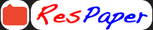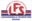Trending ▼   ResFinder# ICSE Class X Prelims 2021 : Mathematics (Lokhandwala Foundation School (LFS), Kandivali East, Mumbai)

4 pages, 32 questions, 20 questions with responses, 27 total responses,20Kiah Jaiswal Lokhandwala Foundation School (LFS), Kandivali East, Mumbai
+Fave Message
 Home > kj_18 >   F Also featured on: School Page icseFormatting page ...

LOKHANDWALA FOUNDATION SCHOOL PRELIMINARY EXAMINATION-(2020-21) SUBJECT :MATHEMATICS Date :25 /01/21 Duration:2 Hrs. Grade: 10 Max. Marks: 80 ____________________________________________________________________________________________ General Instructions:-Attempt all questions from Section A and any four questions from Section B. All working, including rough work, must be clearly shown and must be done on the same sheet as the rest of the answer. Omission of essential working will result in the loss of marks. The intended marks for questions or parts of questions are given in brackets ( ). The paper consists of 4 printed sides. ____________________________________________________________________________________________ SECTION A (40 MARKS) (Attempt all questions) Question.1 a) Mrs. Roy opened a Recurring Deposit Account in a bank. She deposited 2500 per month for two years. At the time of maturity, she received 67500. Find (i)Total interest earned by her. (ii)Rate of interest per annum. b) If A = [ ] and B = [ ] , find the value of 3A - 2B + I , where I is the identity matrix. c) Given that A = {x : 3 < 2x 1 < 9, x R} and B = {x : 11 3x + 2 23, x Represent A and B on number lines. Also, write A (3) (3) R} . B. Question.2 a) KLMN is a quadrilateral , PQ is a tangent to the circle at K. If LN is a diameter of the circle, KLN= 30 and MNL= 60 , find (i) QKN (ii) PKL (iii) MLN (4) (3) b) Manufacturer sold a TV set to the wholesaler for 7000. Wholesaler sold it to the trader at a profit of 1000. If the trader sold it to the customer at a profit of 1500, find (i)Total GST at the rate of 5% collected by State Govt. (ii) Amount that the customer paid for the TV set. (3) c) In the adjoining figure, MN ll QR, PM : MQ = 5 : 4. Find: (i)MN : QR (ii)area( MON) : area( ROQ) (4) Question.3 a) Solve the equation 3x x 7 = 0 and give your answer correct to two decimal places. b) Using factor theorem, show that (x + 4) is a factor of 2x + 9x + x 12. Hence, factorize the given expression completely. (3) (3) P.T.OFormatting page ...

Top Contributors
to this ResPaper
(answers/comments)Shriya Karlapudi(9)Deepali Pawar(6)Svanik Srinivas(4)Parepalli viswambhar(4)Formatting page ...Formatting page ...© 2010 - 2021 ResPaper. Terms of ServiceContact Us Advertise with us

ICSE Q&A - Ask and Answerkj_18 chat